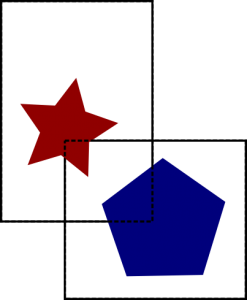### Video game collisions – pixel perfect

Collisions abound in video games. If not, how would you know whether you’ve hit a wall, your enemy, or if that bullet shot you or not? Here we will look at collisions in 2D games, which tend to use sprites (alternatively you can have 3D models projected onto a 2D plane, which is becoming increasingly common).

By virtue of how graphics are stored in computers, the full sprites are rectangular (and some pixels are either alpha pixels or have a special color defined to be transparent – alternatively one can use masks).

Let us then look at collision between rectangles.

Box collision

Consider a rectangle A defined by its vertices$\lbrace (x_{1}^{\text{A}}, y_{1}^{\text{A}}), (x_{1}^{\text{A}}, y_{2}^{\text{A}}), (x_{2}^{\text{A}}, y_{1}^{\text{A}}), (x_{2}^{\text{A}}, y_{2}^{\text{A}}) \rbrace$ and a second rectangle B, also defined by its vertices$\lbrace (x_{1}^{\text{B}}, y_{1}^{\text{B}}), (x_{1}^{\text{B}}, y_{2}^{\text{B}}), (x_{2}^{\text{B}}, y_{1}^{\text{B}}), (x_{2}^{\text{B}}, y_{2}^{\text{B}}) \rbrace$:

It is a simple exercise to calculate the overlap in the x and y directions between the two rectangles to be given by:$x_\text{overlap} = \max(0, \min(x_{2}^{\text{A}}, x_{2}^{\text{B}}) - \max(x_{1}^{\text{A}}, x_{1}^{\text{B}}) )$$y_\text{overlap} = \max(0, \min(y_{2}^{\text{A}}, y_{2}^{\text{B}}) - \max(y_{1}^{\text{A}}, y_{1}^{\text{B}}) )$

where$\max(a,b)$,$\min(a,b)$ are the function that return the largest/smallest of the two values,$a$ or$b$.

Then for our purposes, there is a collision between the rectangles whenever both$x_\text{overlap} \geq 0$ and$y_\text{overlap} \geq 0$.

Pixel perfect

So what about pixel perfect collisions, i.e. when we detect exactly whether or not two sprites have collided?

boxes_objectsSprites and bounding boxes – in this case the two objects do not intersect, although looking only at the bounding box they appear to intersect.

Well, here’s a possible algorithm:

• Detect collisions as in the box collision algorithm above
• If a collision is detected, extract coordinates of collision rectangle
• Iterate over pixels in the collision rectangle: look if there’s any pixel where the sprites have both a non-alpha pixel (or where both masks have the same value, if using masking)
• If any such pixel is found there’s a collision, if none is found, there’s no collision

At most we would have to iterate over the size of one sprite. Let’s estimate that as 300 x 300 = 90.000 pixel, which is still quite a lot, specially if we have to detect a lot of such collisions. But if we look only, for example, at every 10 pixels, in every direction, that would mean iterating at most through 900 pixels, which is 2 orders of magnitude better, considerably faster and nobody can detect that! Although not a pixel perfect collision, it’s much better than box collision and much faster than pixel perfect collisions.